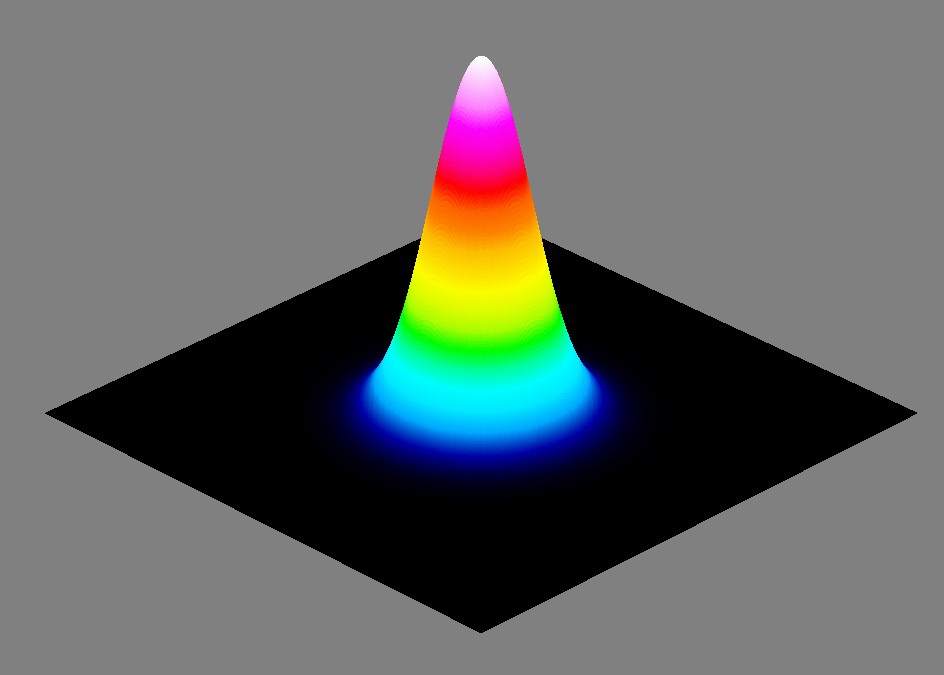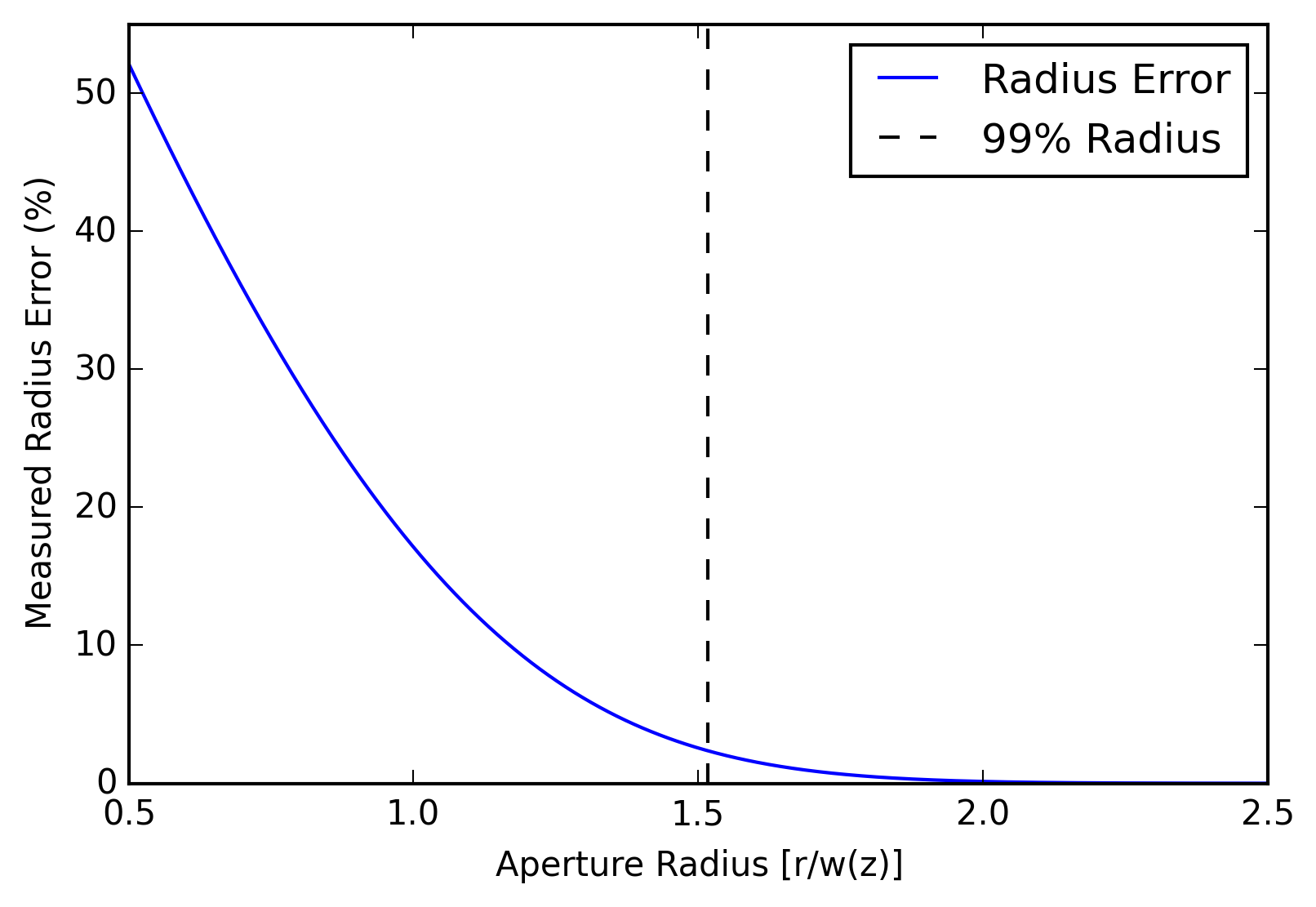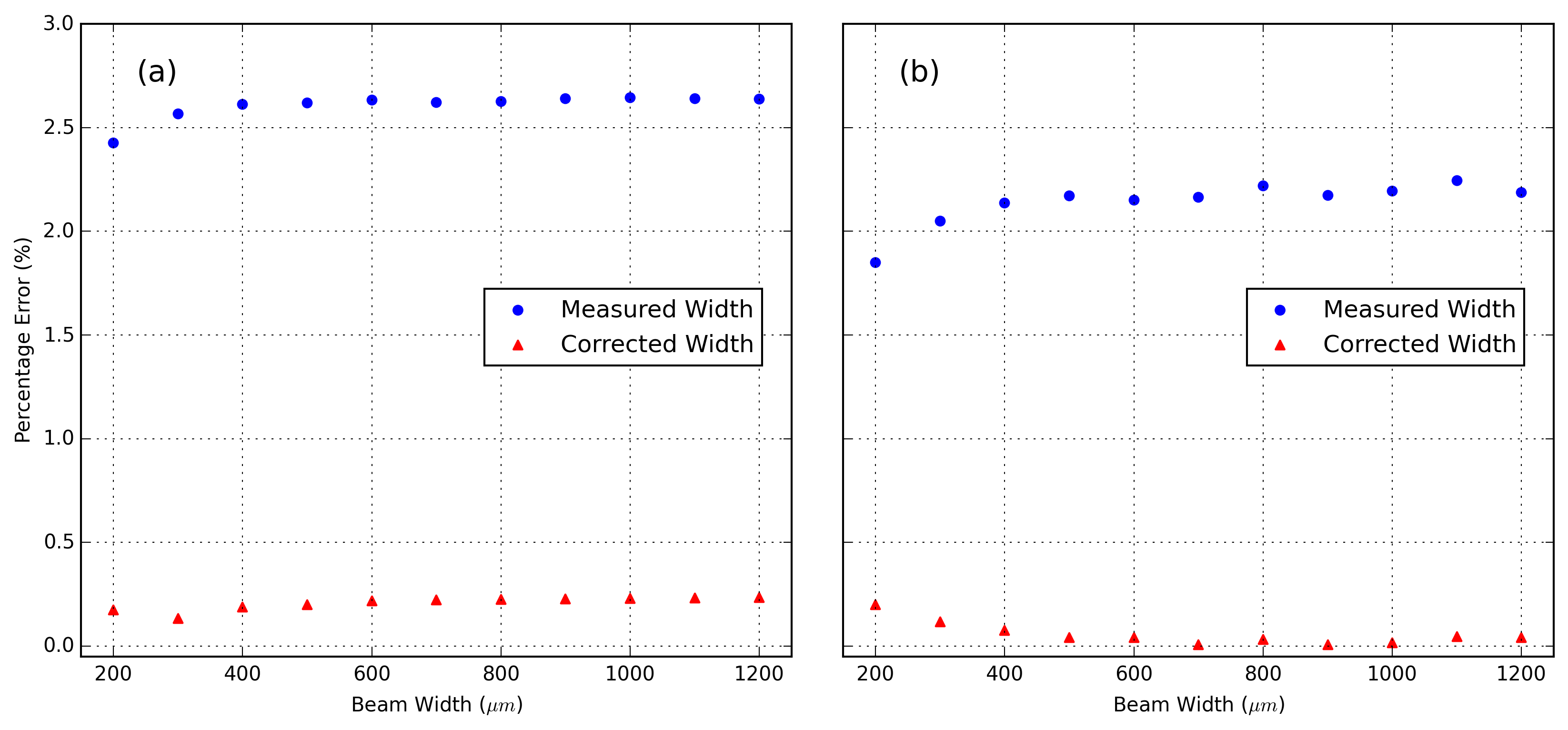May 12, 2016

# Systematic Error in ISO 11146 Measurements

Systematic error is an important calculation in any measurement system, and especially in ISO 11146 laser beam profiling measurements. In this blog post, we address the systematic error inherent to the measurement of beam width as laid out in the ISO 11146 standard. Additionally, we present the results of testing done with our proprietary algorithm that corrects for systematic errors in the ISO 11146 standard's measurement system.

Generally, error is broken down into two categories: statistical and systematic error. Statistical errors are found by taking multiple samples and calculating the mean and standard deviation of the results. Increasing the number of samples taken will improve the accuracy of the measurement, since the Standard Error of the Mean (SEM) follows $$\frac{1}{\sqrt{N}}$$ , where $$N$$ is the number of samples taken. Statistical error can be dealt with through averaging; however, systematic error represents the error inherent in the measurement system and is constant, regardless of the number of samples taken. This blog posts provides an in-depth analysis on the systematic error inherent in the ISO 11146 standard.

#### ISO 11146 Measurements Data Aperture ErrorFigure 1: Example of a TEM$$_{00}$$ fundamental Gaussian beam.

Although the second moment measurement of beam width is the most accurate method available, approximations in the given integrals contribute to systematic error. An example of the integrals used in the second moment measurement is shown below: \begin{equation} \iint I(x, y) \,dx\,dy \end{equation} The integrals for the second moment beam width measurement nominally have their limits at infinity; however, due to the finite area of the sensor surface, the integrals in practice require finite limits. We use a TEM$$_{00}$$ fundamental Gaussian beam as an example. The TEM$$_{00}$$ Gaussian beam is a commonly desired laser output. This theoretical beam serves as a reference beam against which actual beams are compared to in order to determine their quality. The equation for the beam irradiance is given by \begin{equation} I(r, z)= I_{0}e^{-\frac{2}{w^2(z)}r^2} \end{equation} Where $$w(z)$$ is the beam radius when the intensity has fallen to 13.5% of the peak, $$I_0$$ is the max irradiance, and $$r=\sqrt{x^2+y^2}$$ is the radial position. We see that second moment integrals are dependent on the radius, and that the beam technically extends to infinity. However, since the irradiance is a decaying exponential function, it displays asymptotic behavior—it tends towards zero quickly—and the sensor need not extend to infinity. Rather, the integral should include a majority of the beam's power.Figure 2: Measured beam radius percentage error as a function of the aperture width. The radius error (solid) displays decaying exponential behavior. The radius (dotted) corresponding to 99% of a TEM$$_{00}$$ Gaussian's included power has a 2.35% error.

We can find an analytic solution relating the error in the measured radius to the finite limit $$r$$ of the second moment integral (see Figure 2). The second moment is very sensitive to noise. A noisy signal can render a beam measurement unusable. The ISO Standard recommends the use of baselining and thresholding to remove a substantial portion of the noise. An additional software aperture—which includes 99% of the power incident on the sensor—is used to remove the remaining noise. However, if the noise remaining after thresholding is a negligible amount of the 1% power cutoff, then a significant portion of the cutoff is comprised of the beam itself; thus, the accuracy of the second moment beam width measurement is reduced. The aperture radius (i.e., the finite limit $$r$$ corresponding to the 99% inclusion for the TEM$$_{00}$$ beam is $$1.52w(z)$$). This value in conjunction with the analytical expression for beam width error places an upper bound of 2.35% on the error. DataRay has developed a correction factor that accounts for this error in the effective software aperture.

#### DiscretizationFigure 3: (a) Percentage error as a function of the beam width for TEM$$_{00}$$ Gaussian beams without noise. The measured width (circles) had an average error of 2.61% percent, while the corrected with (triangles)—utilizing DataRay's correction factor—had an average error of 0.21%. (b) Percentage error as a function of the beam width for TEM$$_{00}$$ Gaussian beams with Gaussian noise. The Gaussian noise had an average of 2% of the beam's peak power, and a standard deviation of 0.2%. The measured width (circles) had an average error of 2.14%, while the corrected width (triangles) had an average error of 0.06%.

In addition to error from the inclusion region, the discretization of the pixels on the camera sensor array contributes to systematic error. Although the second moments are given as integrals in the ISO 11146 standard, due to the finite number of pixels on the sensor the integrals are in practice Riemann sums. Riemann sums have inherent error, which DataRay's correction factor also accounts for; however, we save an in-depth discussion of discretization for a future blog post.

#### Testing

To determine the effectiveness of our ISO 11146 correction factor we tested a variety of computer generated TEM$$_{00}$$ Gaussian beams in the DataRay software, both with and without noise (see Figure 3). We found that DataRay software's correction factor provides a substantial improvement to the measured beam's width. During testing of perfect Gaussian beams, we found the average measured error to be 2.61% for widths of 20 pixels covered or greater. With DataRay's ISO 11146 Correction Factor, the error was reduced to 0.21%—providing a much higher degree of accuracy. Next, we added Gaussian noise to the Gaussian beams and repeated the tests. With the noisy Gaussian beams the correction factor performed equally well and the initial average error of 2.14% was reduced to 0.06% by our correction factor.

#### DataRay Cameras

All of DataRay's cameras have the ISO 11146 correction factor option in their software. For a strict ISO 11146 beam width or M² measurement, the correction factor can be turned off. However, our research has indicated our correction factor adds a significant degree of accuracy to the beam width measurement. If you have any questions about our beam profiling software or our ISO 11146 correction factor, please contact us.

Posted by: Lucas Hofer